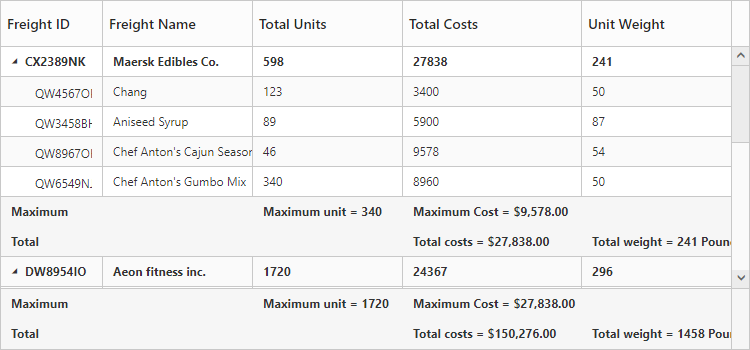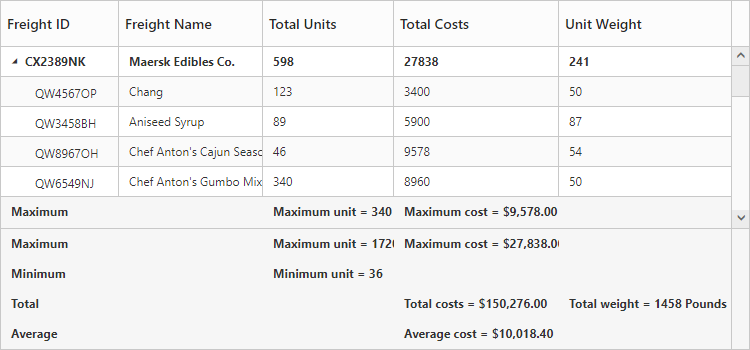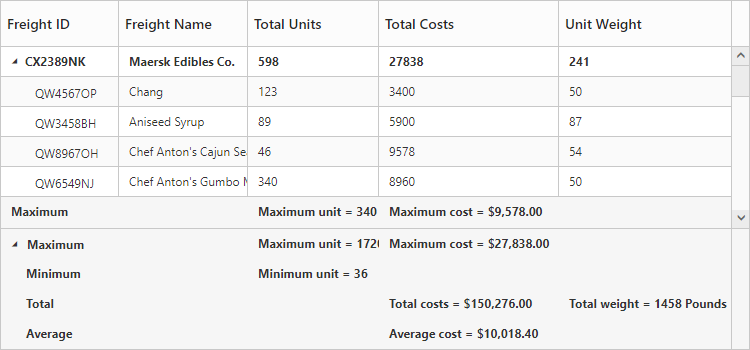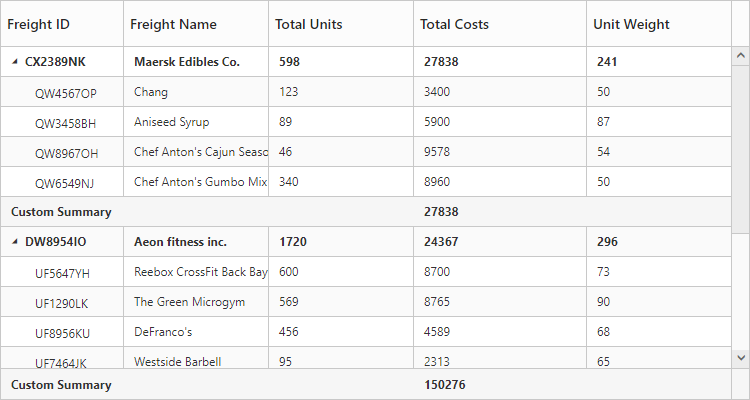# Summary Row

24 Sep 20187 minutes to read

Summary rows in TreeGrid are used to summarize every hierarchy with the set of predefined summary types using the column values. Using the `SummaryRows` property, user can define the summary rows in TreeGrid and the title for each summary row can be defined using the `SummaryRows.Title` property. And summary for the columns in TreeGrid can be defined by using the `SummaryRows.SummaryColumns` property. Summary rows are enabled by setting the `ShowSummaryRow` property as `true`. The total summary row is the overall summary row displayed for all the rows in the TreeGrid and its visibility can be defined by using the `ShowTotalSummary` property.

### Defining summary columns

Using the SummaryType property, user can define the type of summary to be displayed in a column.
The DataMember property is used the map the field values which is used for summary calculations.
The DisplayColumn property is used to specify the column in which the summary to be displayed.
The Prefix and Suffix properties are used to define the text should be displayed along with the summary column value.
The Format property is used for formatting the summary column value.
The below code snippet explains defining a summary row in TreeGrid,

• CSHTML
• ``````@(Html.EJ().TreeGrid("TreeGridContainer")
.ShowSummaryRow(true)
.ShowTotalSummary(true)
.SummaryRows(rows =>
{
rows.Title("Maximum").SummaryColumns(col =>
{
col.SummaryType(TreeGridSummaryType.Maximum)
.DataMember("TotalUnits")
.Prefix("Maximum unit = ")
col.SummaryType(TreeGridSummaryType.Maximum)
.DataMember("TotalCosts")
.Prefix("Maximum Cost = ")
.DisplayColumn("TotalCosts")
rows.Title("Total").SummaryColumns(summary =>
{
summary.SummaryType(TreeGridSummaryType.Sum)
.DataMember("TotalCosts")
.Prefix("Total costs = ")
.DisplayColumn("TotalCosts")
summary.SummaryType(TreeGridSummaryType.Sum)
.DataMember("UnitWeight")
.Prefix("Total weight = ")
.DisplayColumn("UnitWeight")
})
)
@(Html.EJ().ScriptManager())``````

The below screenshot shows the output of above code example.## Customize height of total summary

Using `TotalSummaryHeight` property we can customize the height of the total summary container.
The below code example shows how to update the footer summary container height.

• CSHTML
• ``````@(Html.EJ().TreeGrid("TreeGridContainer")
.ShowSummaryRow(true)
.ShowTotalSummary(true)
.TotalSummaryHeight(120)
)
@(Html.EJ().ScriptManager())``````

The below screenshot shows the output of above code example.## Expand/collapse total summary row

We can expand/collapse the total summary rows in TreeGrid using following methods.

• Using Expander Icon
• Using Method

### Using Expander Icon

We can enable expander icon in total summary row by using `CollapsibleTotalSummary` property. By default expander icon will be rendered in first row of 0th column in total summary rows.
Please find the below code example to enable collapsible total summary row in TreeGrid.

• CSHTML
• ``````@(Html.EJ().TreeGrid("TreeGridContainer")
.ShowSummaryRow(true)
.ShowTotalSummary(true)
.TotalSummaryHeight(120)
.CollapsibleTotalSummary(true)
)
@(Html.EJ().ScriptManager())``````NOTE

We can also customize the expander icon column in total summary row by using `_summaryColumnIndex` property and `Load` event.

### Using Method

Total summary rows in TreeGrid can be expanded/collapsed by using `expandCollapseTotalSummary` method.
Please find the code example to collapse the total summary rows below.

• CSHTML
• ``````<button onclick="expandCollapse()">expandCollapse</button>
@(Html.EJ().TreeGrid("TreeGridContainer")
.ShowSummaryRow(true)
.ShowTotalSummary(true)
.TotalSummaryHeight(120)
.CollapsibleTotalSummary(true)
)
@(Html.EJ().ScriptManager())
function expandCollapse() {
var treeObj = \$("#TreeGridContainer").data("ejTreeGrid");
treeObj.expandCollapseTotalSummary(false);
}``````

Click here to view the demo sample for summary row in TreeGrid.

## Custom Summary

Custom summary can be used to create summary values based on your required custom logic and calculations. To enable the custom summary, the `SummaryType` should be set to ‘Custom’ and the `CustomSummaryValue` property should be defined as function. After the custom calculation, the returned value will be displayed in the corresponding summary cell.

• HTML
• ``````@(Html.EJ().TreeGrid("TreeGridContainer")
.ShowSummaryRow(true)
.SummaryRows(su =>
{
su.Title("Custom Summary").
SummaryColumns(
summary =>
{
summary.SummaryType(TreeGridSummaryType.Custom)
.CustomSummaryValue("sum")
• ``````<script>